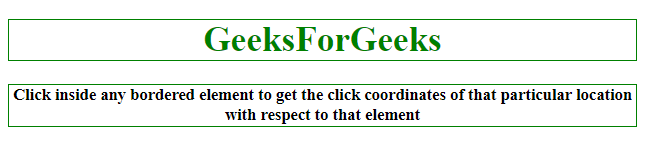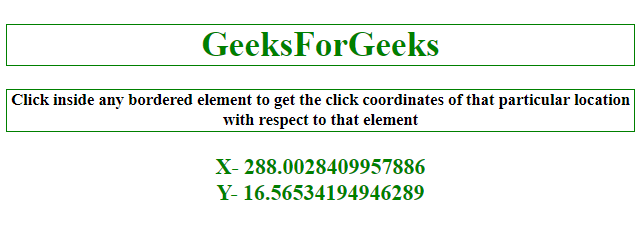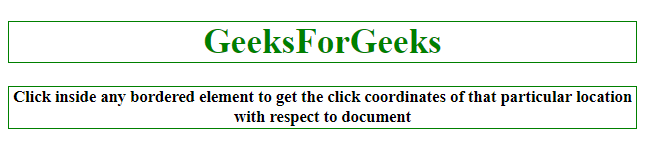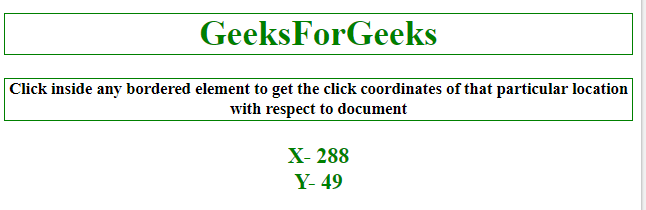# How to get relative click coordinates on the target element using JQuery?

Here is the code to get the coordinates of the element, Here 2 methods are discussed one is calculating the relative position to its parent while another is calculating the relative position to the document.

Approach 1:

• Attach the click event to the element.
• Call an anonymous function when an event occurs.
• Calculate the X relative to the parent element by subtracting the Offset().left property from the pageX property.
• Similarly, calculate the Y relative to parent element by subtracting the Offset().top property from the pageY property.

Example 1: This example follows the approach discussed above to calculate the location’s relative position to its parent element.

 ` ` `<``html``> ` ` `  `<``head``> ` `    ``<``title``> ` `        ``How to get relative click coordinates ` `      ``on the target element using JQuery? ` `    `` ` `    ``<``script` `src``= ` `"https://ajax.googleapis.com/ajax/libs/jquery/3.4.1/jquery.min.js"``> ` `    `` ` `    ``<``style``> ` `        ``h1 { ` `            ``border: 1px solid green; ` `        ``} ` `         `  `        ``#GFG_UP { ` `            ``border: 1px solid green; ` `        ``} ` `         `  `        ``button { ` `            ``border: 1px solid green; ` `        ``} ` `    `` ` ` ` ` `  `<``body` `style``=``"text-align:center;"` `id``=``"body"``> ` `    ``<``h1` `style``=``"color:green;"``>   ` `            ``GeeksForGeeks   ` `        `` ` `    ``<``p` `id``=``"GFG_UP"` `style="font-size: 15px; ` `              ``font-weight: bold;"> ` `    `` ` `    ``<``p` `id``=``"GFG_DOWN"` `style="color:green; ` `              ``font-size: 20px;  ` `              ``font-weight: bold;"> ` `    `` ` `    ``<``script``> ` `        ``var el_up = document.getElementById("GFG_UP"); ` `        ``var el_down = document.getElementById("GFG_DOWN"); ` `        ``var x, y; ` `        ``el_up.innerHTML = "Click inside any bordered element to get the"+  ` `        ``"click coordinates of that particular location with respect"+ ` `        ``"to its parent element "; ` `        ``\$('h1').click(function(e) { ` `            ``// element that has been clicked. ` `            ``var elm = \$(this); ` ` `  `            ``// getting the respective ` `            ``x = e.pageX - elm.offset().left; ` ` `  `            ``// coordinates of location. ` `            ``y = e.pageY - elm.offset().top; ` `            ``gfg_Run(); ` `        ``}); ` `        ``\$('#GFG_UP').click(function(e) { ` `            ``var elm = \$(this); ` `            ``// getting the respective ` `            ``x = e.pageX - elm.offset().left; ` ` `  `            ``// coordinates of location. ` `            ``y = e.pageY - elm.offset().top; ` `            ``gfg_Run(); ` `        ``}); ` ` `  `        ``function gfg_Run() { ` `            ``el_down.innerHTML = "X- " + x + "<``br``>Y- " + y; ` `        ``} ` `    `` ` ` ` ` `  ` `

Output:

• Before clicking on the button:• After clicking on the button:Approach 2:

• Attach the click event to the element.
• Call an anonymous function when event occurs.
• Calculate the X relative to document by pageX property.
• Similarly, calculate the Y relative to document by pageY property.

Example 2: This example follows the approach discussed above to calculate the location’s relative position to the document.

 ` ` `<``html``> ` ` `  `<``head``> ` `    ``<``title``> ` `        ``How to get relative click coordinates ` `      ``on the target element using JQuery? ` `    `` ` `    ``<``script` `src``= ` `"https://ajax.googleapis.com/ajax/libs/jquery/3.4.1/jquery.min.js"``> ` `  `` ` `    ``<``style``> ` `        ``h1 { ` `            ``border: 1px solid green; ` `        ``} ` `         `  `        ``#GFG_UP { ` `            ``border: 1px solid green; ` `        ``} ` `         `  `        ``button { ` `            ``border: 1px solid green; ` `        ``} ` `    `` ` ` ` ` `  `<``body` `style``=``"text-align:center;"` `id``=``"body"``> ` `    ``<``h1` `style``=``"color:green;"``>   ` `            ``GeeksForGeeks   ` `        `` ` `    ``<``p` `id``=``"GFG_UP"` `       ``style="font-size: 15px;  ` `              ``font-weight: bold;"> ` `    `` ` `    ``<``p` `id``=``"GFG_DOWN"`  `       ``style="color:green; ` `              ``font-size: 20px;  ` `              ``font-weight: bold;"> ` `    `` ` `    ``<``script``> ` `        ``var el_up = document.getElementById("GFG_UP"); ` `        ``var el_down = document.getElementById("GFG_DOWN"); ` `        ``var x, y; ` `        ``el_up.innerHTML = "Click inside any bordered element "+ ` `          ``"to get the click coordinates of that particular "+ ` `          ``"location with respect to document"; ` `        ``\$('h1').click(function(e) { ` `           `  `          ``// element that has been clicked. ` `            ``var elm = \$(this);  ` `           `  `          ``// getting the respective ` `            ``x = e.pageX;  ` `           `  `          ``// coordinates of location. ` `            ``y = e.pageY;  ` `            ``gfg_Run(); ` `        ``}); ` `        ``\$('#GFG_UP').click(function(e) { ` `            ``// element that has been clicked. ` `            ``var elm = \$(this); ` ` `  `            ``// getting the respective ` `            ``x = e.pageX; ` ` `  `            ``// coordinates of location. ` `            ``y = e.pageY; ` `            ``gfg_Run(); ` `        ``}); ` ` `  `        ``function gfg_Run() { ` `            ``el_down.innerHTML = "X- " + x + "<``br``>Y- " + y; ` `        ``} ` `    `` ` ` ` ` `  ` `

Output:

• Before clicking on the button:• After clicking on the button:Whether you're preparing for your first job interview or aiming to upskill in this ever-evolving tech landscape, GeeksforGeeks Courses are your key to success. We provide top-quality content at affordable prices, all geared towards accelerating your growth in a time-bound manner. Join the millions we've already empowered, and we're here to do the same for you. Don't miss out - check it out now!

Previous
Next